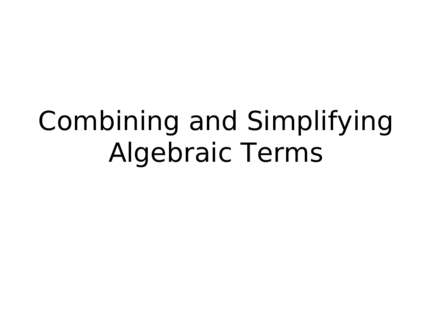# Combining and Simplifying Algebraic ExpressionsContributed by:This pdf explains how to combine the like terms in algebraic expressions and simplify them with examples for better understanding.
1. Combining and Simplifying
Algebraic Terms
2. Warm Up
Simplify each expression
1. a²+2b+2a²+b+2c
2. 5x+6x²+3y+2x+8y+9x²
3. g+h+2g+5h+8g²
3. 7x + 4
Constant - a
• Coefficient – a
number that cannot
number in front of a
change
variable. This number
is multiplied by the
variable.
Variable – a letter or
symbol that represents an
unknown number.
4. x y x² a r b²
• Variables are similar to species of animals.
• Different species of animals can’t mate and
produce (fertile) off spring.
A dog and cat can’t mate and produce
a dat.
5. x y x² a r b²
To be able to combine like terms, the
terms must:
1. Be the same variable
2. Have the same exponent
3b + 4c + 2b = 5b +4c
4x²-3c
x² - 3c + 3x² =
6. z= p= g=
Combine the like terms
A. 4z + 6 g + 2p + 3z + 5p + 1g
4z + 3z = 7z
Combine all the like terms
2p + 5p = 7p in a new algebraic
expression:
6g + 1g = 7g
7z +7p + 7g
7. c= p= g=
Combine the like terms
A. 10c + 4 g + 5p + 15c – 12 p - 3g
10c + 15 c = 25c
5p +(-12p) = -7p Combine all the like terms
in a new algebraic
4g + (-3g) = 1g
expression:
25c + (-7p) +1g
8. s= p= w=
Combine the like terms
A. -4s - 6w + 8p + 12s – 10 p + 13w
-4s + 12s = 8s
8p + (-10p) = -2pCombine all the like terms
in a new algebraic
-6w + 13w = 7w
expression:
8s + (-2p) + 7w
9. z= b= x=
• Use the distributive property and combine
the like terms Combine 5x + 3x = 8x and
• 3(4z + 5x + 6b + 3x) rewrite the algebraic expression.
3(4z) = 3x4 = 12z
• 3(4z + 8x + 6b)
3(8x) = 3X8 = 24x
3(6) = 18b
12z + 24x + 18b
10. X= X²= y=
Combine the like terms
5x + 7x² -y + 4x – 15x² + 4y + x²
5x + 4x = 9x
7x² + (-15x²) + x² = -7x²
-y + 4y = 3y Combine all the like terms
in a new algebraic
expression:9x + (-7x²) + 3y
Or 9x -7x²+3y
11. X= X²= y= y²= p=
Combine the like terms:
5x y² 4x² 6y 3p 8x -9y² -3y 2x
5x + 8x + 2x = 15x
y² + (-9y²) = -8y²
6y + (-3y) = 3y
Combine all the like terms in a new
algebraic expression:
15x - 8y² +3y + 4x² + 3p